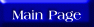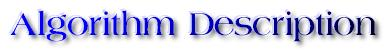The heapsort algorithm uses the data structure called the heap. A heap is defined as a complete binary tree in which each node has a value greater than both its children (if any). Each node in the heap corresponds to an element of the array, with the root node corresponding to the element with index 0 in the array. Considering a node corresponding to index i, then its left child has index (2*i + 1) and its right child has index (2*i + 2). If any or both of these elements do not exist in the array, then the corresponding child node does not exist either. Note that in a heap the largest element is located at the root node. The code for the algorithm is:

Heapify(A, i){
l <- left(i)
r <- right(i)
if l <= heapsize[A] and A[l] > A[i]
then largest <- l
else largest <- i
if r <= heapsize[A] and A[r] > A[largest]
then largest <- r
if largest != i
then swap A[i] <-> A[largest]
Heapify(A, largest)
}

Buildheap(A){
heapsize[A] <- length[A]
for i <- |length[A]/2| downto 1
do Heapify(A, i)
}

Heapsort(A){
Buildheap(A)
for i <- length[A] downto 2
do swap A <-> A[i]
heapsize[A] <- heapsize[A] - 1
Heapify(A, 1)
}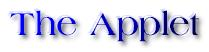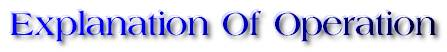Heap Sort operates by first converting the initial array into a heap. The HeapSort algorithm uses a process called Heapify to accomplish its job. The Heapify algorithm, as shown above, receives a binary tree as input and converts it to a heap. The root is then compared with its two immediate children, and the larger child is swapped with it. This may result in one of the left or right subtrees losing their heap property. Consequently, the Heapify algorithm is recursively applied to the appropriate subtree rooted at the the node whose value was just swapped with the root and the process continues until either a leaf node is reached, or it is determined that the heap property is satisfied in the subtree.

The entire HeapSort method consists of two major steps:

STEP 1: Construct the initial heap using adjust (N/2) times on all non-leaf nodes.
STEP 2: Sort by swapping and adjusting the heap (N-1) times.

In step 2, since the largest element of the heap is always at the root, after the initial heap is constructed, the root value is swapped with the lowest, rightmost element. This node corresponds to the last element of the array and so after the swapping the righmost element of the array has the largest value, which is what we want it to have. Consequently, the rightmost element is correctly place, and so the corresponding node becomes a light gray in the tree display depicting the heap. Also, the elements (nodes) chosen for swapping at this point of the algorithm are shown as yellow nodes.

Thereafter, since the value of the lowest, rightmost node has moved up to the root, the heap property of the rest of the heap (minus the gray nodes) has been disturbed. Consequently, now the Heapify process is applied to the rest of the heap (treating the light gray nodes as though they were no longer a part of the heap). Once the rest of the heap is again converted to a proper heap, the swapping process as described in the previous paragraph, followed by the Heapify process is applied again. This continues until the root node of the heap turns light gray - i.e., the root has its correct value. Then, the array is sorted.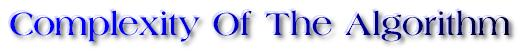At each step of the Heapify algorithm, a node is compared to its children and one of them is chosen as the next root. One level of the tree is dropped at each step of this process. Since the heap is a complete binary tree, there are atmost log(N) levels. Thus, the worst case time complexity of Heapify is O(log(N)). In the Heapsort algorithm, Heapify is used (N/2) times to construct the initial heap and (N-1) times to sort. Thus the Heapsort algorithm has a worst case time complexity of O(N*log(N)).